Courses

# Test: Limit With Solution (Competition Level) - 2

## 30 Questions MCQ Test Mathematics For JEE | Test: Limit With Solution (Competition Level) - 2

Description
This mock test of Test: Limit With Solution (Competition Level) - 2 for JEE helps you for every JEE entrance exam. This contains 30 Multiple Choice Questions for JEE Test: Limit With Solution (Competition Level) - 2 (mcq) to study with solutions a complete question bank. The solved questions answers in this Test: Limit With Solution (Competition Level) - 2 quiz give you a good mix of easy questions and tough questions. JEE students definitely take this Test: Limit With Solution (Competition Level) - 2 exercise for a better result in the exam. You can find other Test: Limit With Solution (Competition Level) - 2 extra questions, long questions & short questions for JEE on EduRev as well by searching above.
QUESTION: 1

###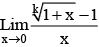(k is a positive integer)

Solution:

Using – L- Hospital rule

QUESTION: 2

###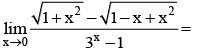Solution:

On rationalizing

QUESTION: 3

###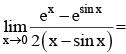Solution: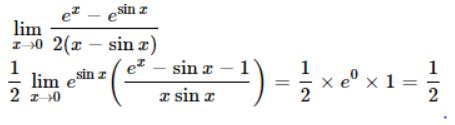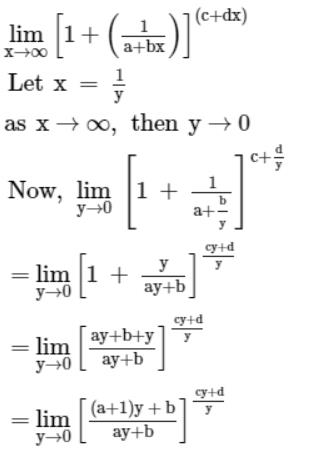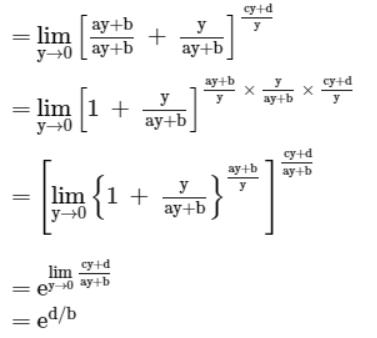QUESTION: 4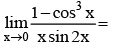Solution:

Given limit is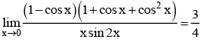QUESTION: 5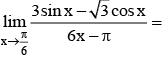Solution:

By L- Hospital rule

QUESTION: 6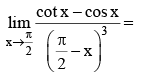Solution: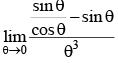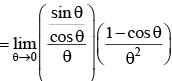QUESTION: 7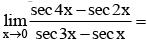Solution:

Divide numerator and denominator by x2

QUESTION: 8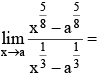Solution:

Using L hospital rule
lim x-->a d/dx[(x - a^⅝)/(x - a)
lim x-->a   [5/8(x-⅜)]/1/3(x-⅔)
lim x-->a 15/8 [x]/[x]
lim x-->a  15/8 [x⅔-⅜]
Limx-->a  15/8 [x7/24]

QUESTION: 9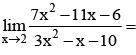Solution:

Using L-Hospital rule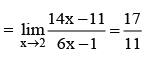QUESTION: 10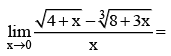Solution:

Using L-Hospital rule

QUESTION: 11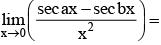Solution:

Using L- Hospital Rule

QUESTION: 12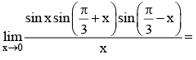Solution:

lim(x→0) sinx sin(π/3+x) sin(π/3−x)x
= lim(x→0) (sinx/x) sin(π/3+x) sin(π/3−x)
= 1 (sin(π/3) (sin(π/3)
= 1(√3/2)(√3/2)
= 3/4

QUESTION: 13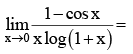Solution: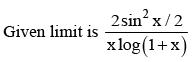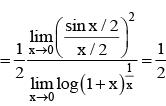QUESTION: 14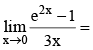Solution:

Using L-Hospital rule

QUESTION: 15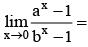Solution:

Use L- Hospital Rule

QUESTION: 16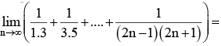Solution:

Given limit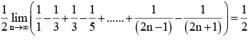QUESTION: 17

If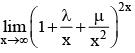= e2 then

Solution:

Given limit is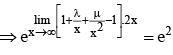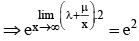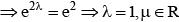QUESTION: 18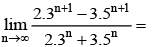Solution: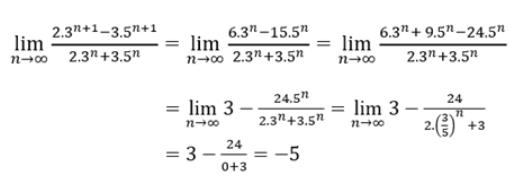QUESTION: 19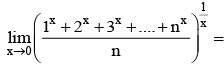Solution:

(1.2.3.....n)1/n

QUESTION: 20

If [x] denotes the greatest integer less than or equal to x then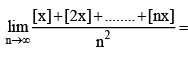Solution:

By using Sandwitch theorem

QUESTION: 21

Let f : R → R be a positive increasing function with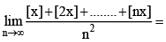Solution:

[x] can be written as X+{x}    --> {x} is fractional part .. between 0 and 1
therefore ,
lim n→∞ {[x]+[2x]+[3x]+.........+[nx]}/n2
is = lim n→∞  {x+2x+3x+.........+nx + {x}+{2x} ...+{nx}}/n2
= {x(1+2+3+..+n)   +   {x}+...{nx}}/n2
= x(n(n+1))/2n2 + ({x}+...{nx})/n2
Since {} is only b/w 0 and 1  , the second operand becomes 0 as n tends to ∞
on solving the first part , u get (n2x +nx)/2n2 = x/2 + x/2n
x/2n becomes 0 as x tends to infinity.
therefore the answer is x/2.

QUESTION: 22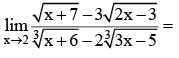Solution:

Using L- hospital rule

QUESTION: 23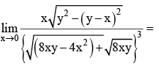Solution:

Take common higher power of x in both numerator and denominator

QUESTION: 24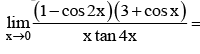Solution:

limx→0 [(1−cos2x)(3+cosx)]/xtan4x
= limx→0(1-(1-2sin^2x))(3+cosx)/xtan4x
= limx→0 (2sin/xx)(sinx)(3+cosx)/tan4x
= lim x→0 (2sinx/x)(sinx)(3+cosx)/(4x(tan4x/4x))
= limx→0(2sinxx)(sinx)3+cosx4xtan4x4x
We know, limx→0 sinx/x=1 and limx→0 tanx/x=1
∴ our expression becomes,
= 2/4 limx→0 (3+4cosx)
= 2/4limx→0 (3+4cosx)
As cos0=1
∴ our expression becomes, =2/4(3+1) = 2

QUESTION: 25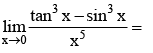Solution: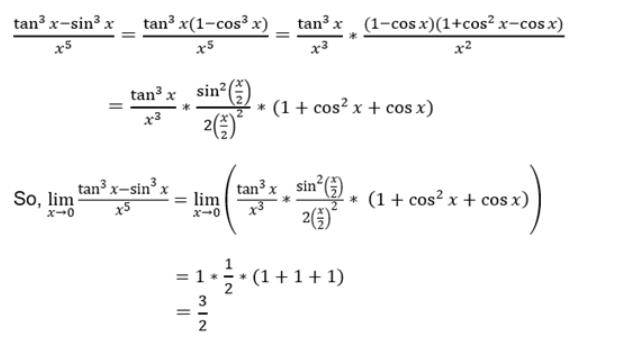QUESTION: 26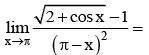Solution: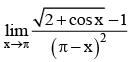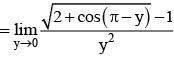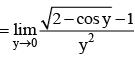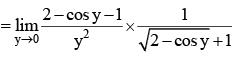QUESTION: 27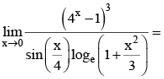Solution:

Using standard formulae

QUESTION: 28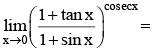Solution:

1 form

QUESTION: 29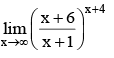Solution:

e6-1 = e5

QUESTION: 30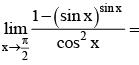Solution:

Use L- Hospital rule